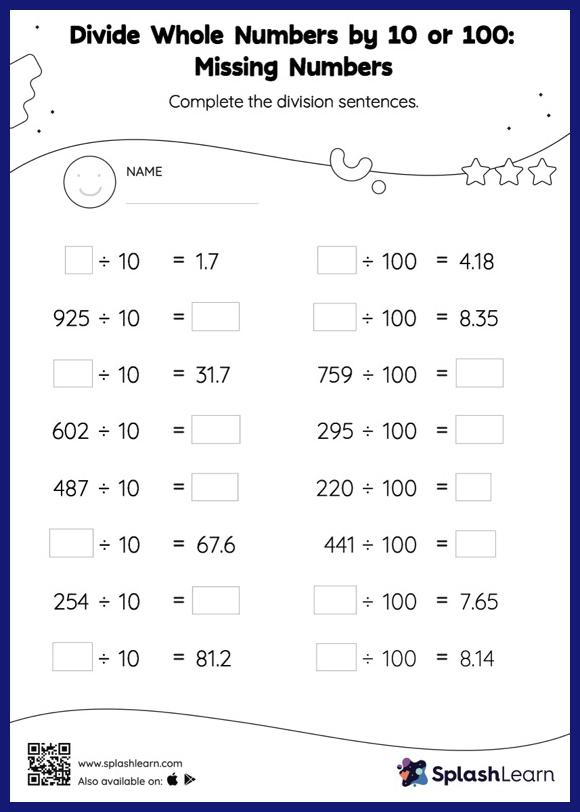# Divide Whole Numbers by 10 or 100: Missing Numbers Worksheet

Home > Divide Whole Numbers by 10 or 100: Missing NumbersHelp your little one develop a knack for math with this divide whole numbers by 10 or 100 worksheet. Dividing a number by 10 moves each digit one place to the right, and dividing by 100 moves each digit two places to the right. Learners practice this concept extensively in divide whole numbers by 10 or 100 worksheet as they find the missing number in decimal division. In each problem, the numbers are laid out in the horizontal format. Students should try to use different strategies involving composing and decomposing numbers to solve these problems. This will help them develop flexibility and fluency.